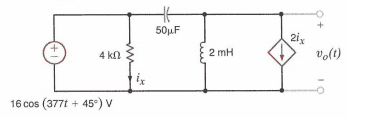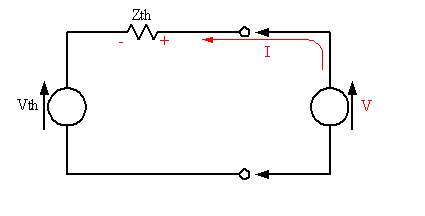# Thevenins equivalent for an AC circuit

• Engineering
• stef6987

## Homework StatementJust before reading, i am posting this as this question seemed way to easy to solve in my assignment, problems aren't usually this easy ! haha.
I need to find V0, i originally just found the voltage at that node using KCL which was straight forward, but in my lecture notes the lecturer added a voltage source inbetween the two terminal, but he was trying to find thevenins equiv voltage, my question is, should i be adding the vth (or vo whatever you want to call it) voltage source between the terminals where v0 is and have a current going upwards (ith) in this case and solve for vth or vo? or can i just find the voltage at that node

## The Attempt at a Solution

I just took kcl at the vo node and got this:
V/(j754) + 2(ix) + (V-(16∠45°))/(-j53.05)) = 0?

If i was to add the voltage source between the terminals, my KCL equation would be the same except instead of it equalling 0 it would equal Ith

V/(j754) + 2(ix) + (V-(16∠45°))/(-j53.05)) = Ith

i just need clarification which method is correct, thankyou!

Last edited:

For this circuit you can find the Thevenin voltage as the open circuit potential just fine; the circuit is such that the controlled current source does not depend upon the output voltage (it gets its reference value from a fixed current determined by a resistor and a fixed voltage source). Since the controlled source is really a fixed source in disguise, you can even suppress it to find the Thevenin resistance. However, it's not always the case that these conditions hold for a circuit under analysis.

The second method, adding a voltage source to the output port and finding the current that it drives into the network, kills two birds with one stone; It allows you to determine both the Thevenin voltage and the Thevenin resistance with the same procedure.

Consider the typical Thevenin model:If voltage source V drives current I into the model, then we can write:

##V = Vth + I\cdot Zth##

Thus, if you stick a voltage source V onto the output of your circuit and solve for V, you should end up with an expression that you can wrangle into the form above, and you can simply pick out the two terms#### Attachments

For this circuit you can find the Thevenin voltage as the open circuit potential just fine; the circuit is such that the controlled current source does not depend upon the output voltage (it gets its reference value from a fixed current determined by a resistor and a fixed voltage source). Since the controlled source is really a fixed source in disguise, you can even suppress it to find the Thevenin resistance. However, it's not always the case that these conditions hold for a circuit under analysis.

The second method, adding a voltage source to the output port and finding the current that it drives into the network, kills two birds with one stone; It allows you to determine both the Thevenin voltage and the Thevenin resistance with the same procedure.

Consider the typical Thevenin model:If voltage source V drives current I into the model, then we can write:

##V = Vth + I\cdot Zth##

Thus, if you stick a voltage source V onto the output of your circuit and solve for V, you should end up with an expression that you can wrangle into the form above, and you can simply pick out the two termsthankyou for the well explained response :)
I only need to find the voltage v0, so i suppose it would be easier to use my original method, if you don't mind checking, is the KCL for my first equation correct? thankyou!

You're welcome. Your KCL looks okay to meYou're welcome. Your KCL looks okay to meI attempted both methods just to ensuer my answer was correct, by adding a voltage source i got my V0 in the form of V0 = -56.92j*Ith + (12.43j + 11.79)

without using the source i just got 12.43j + 11.79 which makes sense because I'm just finding Vth in this case. I think i will go with the form of V0 = -56.92j*Ith + (12.43j + 11.79)

What do you think?

thanks :)

Have you misplaced a decimal point in the inductor impedance? The inductance is given as 2 mH, so its impedance should be 0.754j Ω. The reason I ask is that your result values look a little high.

Yes i just realized haha, thankyou. Now when i get the Voltage in the form of I.Z + V, can i just use voltage division? the voltage where trying to find being the voltage across the 2mH inductor?

Yes i just realized haha, thankyou. Now when i get the Voltage in the form of I.Z + V, can i just use voltage division? the voltage where trying to find being the voltage across the 2mH inductor?

The voltage across the inductor is the same voltage as Vo. If a load impedance is placed on the output then sure, a voltage divider is formed.

The voltage across the inductor is the same voltage as Vo. If a load impedance is placed on the output then sure, a voltage divider is formed.

I just used a simple KCL, seemed like a more simple answer, thankyou so much for your help, it's very appreciated :)